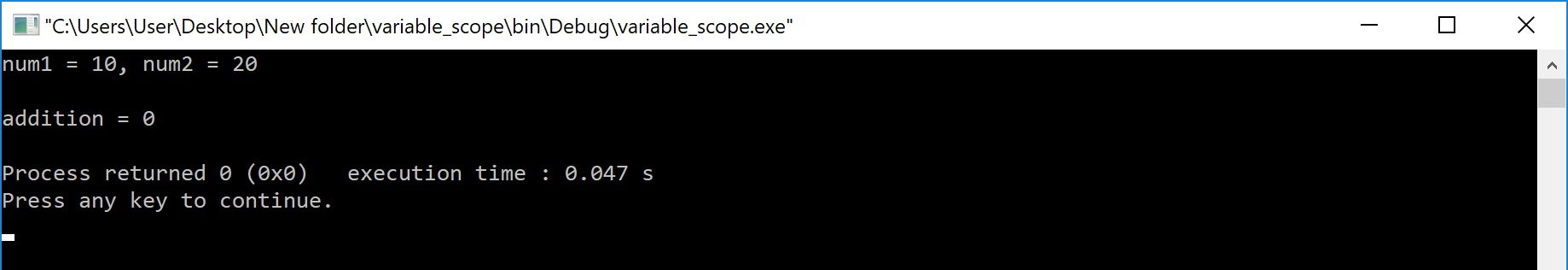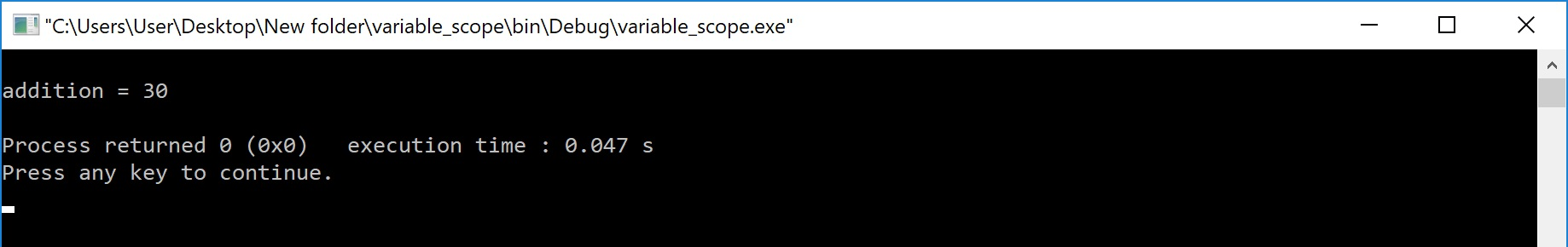The perfect place for easy learning...

×

# Scope of Variable in C

When we declare a variable in a program, it can not be accessed against the scope rules. Variables can be accessed based on their scope. The scope of a variable decides the portion of a program in which the variable can be accessed. The scope of the variable is defined as follows...

Scope of a variable is the portion of the program where a defined variable can be accessed.

The variable scope defines the visibility of variable in the program. Scope of a variable depends on the position of variable declaration.

In C programming language, a variable can be declared in three different positions and they are as follows...

• Before the function definition (Global Declaration)
• Inside the function or block (Local Declaration)
• In the function definition parameters (Formal Parameters)

# Before the function definition (Global Declaration)

Declaring a variable before the function definition (outside the function definition) is called global declaration. The variable declared using global declaration is called global variable. Tha global variable can be accessed by all the functions that are defined after the global declaration. That means the global variable can be accessed any where in the program after its declaration. The global variable scope is said to be file scope.

# Example Program

``````#include<stdio.h>
#include<conio.h>

int num1, num2 ;
void main(){
void subtraction() ;
void multiplication() ;
clrscr() ;
num1 = 10 ;
num2 = 20 ;
printf("num1 = %d, num2 = %d", num1, num2) ;
subtraction() ;
multiplication() ;
getch() ;
}
{
int result ;
result = num1 + num2 ;
}
void subtraction()
{
int result ;
result = num1 - num2 ;
printf("\nsubtraction = %d", result) ;
}
void multiplication()
{
int result ;
result = num1 * num2 ;
printf("\nmultiplication = %d", result) ;
}```
```

Output:In the above example program, the variables num1 and num2 are declared as global variables. They are declared before the main() function. So, they can be accessed by function main() and other functions that are defined after main(). In the above example, the functions main(), addition(), subtraction() and multiplication() can access the variables num1 and num2.

# Inside the function or block (Local Declaration)

Declaring a variable inside the function or block is called local declaration. The variable declared using local declaration is called local variable. The local variable can be accessed only by the function or block in which it is declared. That means the local variable can be accessed only inside the function or block in which it is declared.

# Example Program

``````#include<stdio.h>
#include<conio.h>

void main(){
int num1, num2 ;
clrscr() ;
num1 = 10 ;
num2 = 20 ;
printf("num1 = %d, num2 = %d", num1, num2) ;
getch() ;
}
{
int sumResult ;
sumResult = num1 + num2 ;
}```
```

Output:The above example program shows an error because, the variables num1 and num2 are declared inside the function main(). So, they can be used only inside main() function and not in addition() function.

# In the function definition parameters (Formal Parameters)

The variables declared in function definition as parameters have a local variable scope. These variables behave like local variables in the function. They can be accessed inside the function but not outside the function.

# Example Program

``````#include<stdio.h>
#include<conio.h>

void main(){
int num1, num2 ;
clrscr() ;
num1 = 10 ;
num2 = 20 ;
getch() ;
}
{
int sumResult ;
sumResult = a + b ;